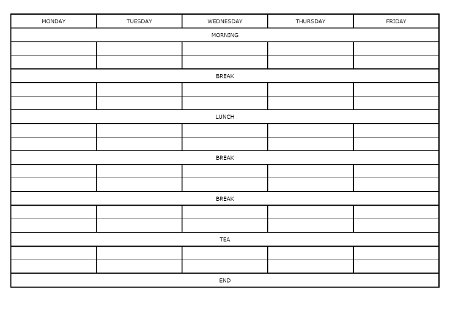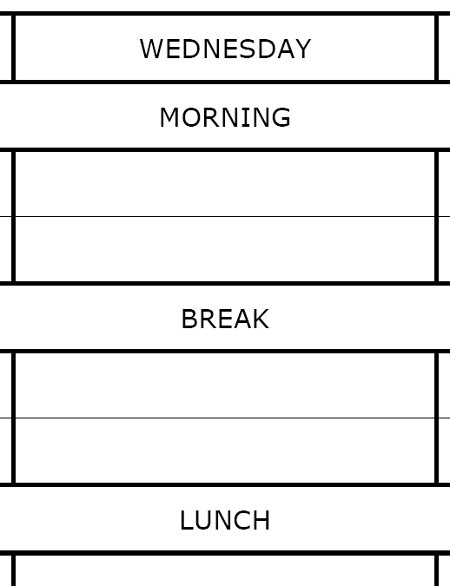# Exam Technique in Physics

## Basics

• Don’t panic.
• Words like ‘calculate’, ‘define’ and ‘state’ have specific meanings; follow these instructions.
• If a calculation question asks you to ‘show’ something (e.g. “Show that the speed of the car is 30 m/s.”) and you can’t get the correct answer, use the answer from the question, not your incorrect answer, in any follow-up questions.
• If you don’t know what to do, do something. Never leave a blank space.
• Don’t make your answers overly long. If a question has space for four lines, don’t be afraid to write five or six; but eight or ten is too many.
• Bullet points are your friend when answering longer-answer questions. A three-mark question needs three bullet points, a four-mark question needs four, and so on.
• Use common sense: if you calculate the speed of a car as a million metres per second or the mass of a person as 800 kg then you have made a mistake.
• Be specific, e.g. nuclear power plants “create nuclear waste” rather than “create pollution”; “air resistance” wastes energy rather than “friction”.
• If you have two questions left to answer but only time to answer one, then do the first half of both; questions generally get harder as you work through them.
• Don’t use terms you don’t understand or that you haven’t actually learnt about, e.g. inertia.
• Don’t go for complicated risky answers – stick with the most basic, simplest, guaranteed-to-be-correct answer, e.g. the risk from nuclear power is “dangerous waste” not “visual pollution”.
• Don’t be afraid to use spare space on the exam paper for a sketch diagram, this can be very helpful in thinking a problem through.
• ## Calculations

• Remember the three-step process:
• Write down the equation you are going to use. If the “standard” form of the equation (e.g. F=ma) needs to be rearranged (e.g. a=F/m) then write down both forms, in case you rearrange the equation incorrectly.
• Substitute the quantities from the question into your formula and carry out the calculation.
• Write down the answer and don’t forget to use the correct unit.
• Always work in standard units: convert centimetres and kilometres to metres, and hours and minutes to seconds before you start answering the question.
• Watch for multipliers and submultipliers: you must be familiar with centi-, milli-, micro-, kilo-  and mega-.
• Use standard form for answers with large numbers of zeroes. It is very easy to accidentally add or remove a zero when writing out 0.000000831 but not in 8.31×10−7.
• Make sure you know how to use your calculator, particularly how to use the calculator’s built in memory and the reciprocal function. Make sure that when doing trigonometry (sin, cos and tan) your calculator is set in degrees mode and not in radians or gradians.
• Don’t write too many significant figures in your answer: three significant figures is almost certainly enough. But make sure that during calculations you use the full answer – storing it in the memory or using the ‘previous answer’ key – to avoid rounding errors.

## Corrections

• Make sure that an examiner can tell which answer is the one you want marked. Cross incorrect work through clearly and make it obvious what your answer is; underlining in a different colour or writing “Answer =” is a good idea.
• Do not use double-headed arrows to swap answers around; examiners may ignore them.

## Graphs

• When drawing a graph make sure you use the majority of the grid space available.
• Always think about the features of the graph: what do the axis intercepts, the gradient of the line and the area underneath the line mean – what is their physical significance?
• When answering graph questions be specific about what you are doing: state “area under graph” or “gradient of line” rather than just showing the calculations themselves.
• When finding the gradient of a line make sure that you use a large portion (at least two-thirds) of the line to create your rise-run triangle.
• Read the axes carefully: is the scale in newtons or kilonewtons, seconds or milliseconds?

## Language

• Use scientific language: “volume is constant” is a much better answer than “container is something that cannot expand”.
• Don’t write “probably” or “possibly” or “about” or “quite”; don’t write “approximately” unless that’s really what you mean.
• Match adjectives to quantities, e.g. “long wavelength” rather than “high wavelength” and “high frequency” rather than “large frequency”.
• Don’t begin an answer with “It” or “Because”, these can create ambiguity in your answer. “The car accelerates …” is much better than “It accelerates …”.
• To paraphrase Einstein: make your answers as simple as possible, but no simpler. There is no requirement for every answer to be a full sentence, but one word answers will often not get you all the available marks.
• Don’t use exclamation marks. Nothing in an exam requires an exclamation mark.

# How to make a revision timetable

If you’re interested in this post, you might also be interested in my post about
exam technique in physics.There’s a running joke amongst teachers about the pupil who spends so much time preparing a revision timetable that they never actually do any revision. Like all good comedy, it’s at least partially based in fact. It’s very easy to allow something – anything – to distract you from your revision.

But this isn’t going to be a problem for you. I’ve made a revision timetable for you.

The timetable has six 1-hour blocks of revision per day. Each 1-hour block is broken into two 25-minute revision sessions separated by a ten minute break. This ten minute break allows you to cool off, and gives you some room to manoeuvre if you’re coming to the end of a topic and you don’t want to run into the next session.

There are five breaks splitting up the six sessions: two 1-hour breaks (for lunch and tea) and three 30-minute breaks.

Let’s take Wednesday as an example:I suggest you start in the morning at 1000 so Session 1 runs from 1000-1025, followed by a ten minute break, then Session 2 from 1035-1100. Session 3 starts after a half-hour break at 1130 and runs ’til 1155, then Session 4 from 1205-1230. Session 5 begins (after a one-hour lunch break) at 1330. The day runs like this until 1930, at which time all revision should stop until the next day.

There are two versions of the revision timetable template to download. One is completely blank and the other has the suggested timing of each session printed on (in light-grey ink so that you can write over it). I suggest you download both.